# 100 AI interview questions and answers for 2024

AI interviews are tough nuts to crack. So, if you are appearing for an AI interview or are about to interview some AI engineers for a vacant position, this list of Artificial Intelligence interview questions and answers will be helpful. It is good to have an idea of what questions to ask or be asked in an AI interview.

Last updated on Nov 28, 2023

As per the latest study, “By 2025, there would be a requirement of 97 million AI professionals in the world to fulfill the rising demands in AI jobs. The industry requires top quality talent who should have extensive knowledge of machine learning, natural language processing, Python programming, cognitive computing, etc.

Therefore, job seekers should be well-prepared with these key concepts because recruiters test all aspects of AI while hiring. Based on those key areas, we have formed a list of top Artificial Intelligence interview questions & answers that will help recruiters and developers in the interview process.

### Basic Artificial Intelligence interview questions and answers

#### 1.

Explain Artificial Intelligence and give its applications.

Artificial Intelligence (AI) is a field of Computer Science focuses on creating systems that can perform tasks that would typically require human intelligence, such as recognizing speech, understanding natural language, making decisions, and learning. We use AI to build various applications, including image and speech recognition, natural language processing (NLP), robotics, and machine learning models like neural networks.

#### 2.

How are machine learning and AI related?

Machine learning and Artificial Intelligence (AI) are closely related but distinct fields within the broader domain of computer science. AI includes not only machine learning but also other approaches, like rule-based systems, expert systems, and knowledge-based systems, which do not necessarily involve learning from data. Many state-of-the-art AI systems are built upon machine learning techniques, as these approaches have proven to be highly effective in tackling complex, data-driven problems.

#### 3.

What is Deep Learning based on?

Deep learning is a subfield of machine learning that focuses on the development of artificial neural networks with multiple layers, also known as deep neural networks. These networks are particularly effective in modeling complex, hierarchical patterns and representations in data. Deep learning is inspired by the structure and function of the human brain, specifically the biological neural networks that make up the brain.

#### 4.

How many layers are in a Neural Network?

Neural networks are one of many types of ML algorithms that are used to model complex patterns in data. They are composed of three layers — input layer, hidden layer, and output layer.

#### 5.

Explain TensorFlow.

TensorFlow is an open-source platform developed by Google designed primarily for high-performance numerical computation. It offers a collection of workflows that can be used to develop and train models to make machine learning robust and efficient. TensorFlow is customizable, and thus, helps developers create experiential learning architectures and work on the same to produce desired results.

#### 6.

What are the pros of cognitive computing?

Cognitive computing is a type of AI that mimics human thought processes.We use this form of computing to solve problems that are complex for traditional computer systems. Some major benefits of cognitive computing are:

• It is the combination of technology that helps to understand human interaction and provide answers.
• Cognitive computing systems acquire knowledge from the data.
• These computing systems also enhance operational efficiency for enterprises.

#### 7.

What’s the difference between NLP and NLU?

Natural Language Processing (NLP) and Natural Language Understanding (NLU) are two closely related subfields within the broader domain of Artificial Intelligence (AI), focused on the interaction between computers and human languages. Although they are often used interchangeably, they emphasize different aspects of language processing.

NLP deals with the development of algorithms and techniques that enable computers to process, analyze, and generate human language. NLP covers a wide range of tasks, including text analysis, sentiment analysis, machine translation, summarization, part-of-speech tagging, named-entity recognition, and more. The goal of NLP is to enable computers to effectively handle text and speech data, extract useful information, and generate human-like language outputs.

While, NLU is a subset of NLP that focuses specifically on the comprehension and interpretation of meaning from human language inputs. NLU aims to disambiguate the nuances, context, and intent in human language, helping machines grasp not just the structure but also the underlying meaning, sentiment, and purpose. NLU tasks may include sentiment analysis, question-answering, intent recognition, and semantic parsing.

#### 8.

Give some examples of weak and strong AI.

Some examples of weak AI include rule-based systems and decision trees. Basically, those systems that require an input come under weak AI. On the other hand, a strong AI includes neural networks and deep learning, as these systems and functions can teach themselves to solve problems.

#### 9.

What is the need of data mining?

Data mining is the process of discovering patterns, trends, and useful information from large datasets using various algorithms, statistical methods, and machine learning techniques. It has gained significant importance due to the growth of data generation and storage capabilities. The need for data mining arises from several aspects, including decision-making.

#### 10.

Name some sectors where data mining is applicable.

There are many sectors where data mining is applicable, including:

Healthcare -It is used to predict patient outcomes, detection of fraud and abuse, measure the effectiveness of certain treatments, and develop patient and doctor relationships.

Finance -The finance and banking industry depends on high-quality, reliable data. It can be used to predict stock prices, predict loan payments and determine credit ratings.

Retail - It is used to predict consumer behavior, noticing buying patterns to improve customer service and satisfaction.

#### 11.

What are the components of NLP?

There are three main components to NLP:

1. Language understanding - This defines the ability to interpret the meaning of a piece of text

2. Language generation - This is helpful in producing text that is grammatically correct and conveys the intended meaning.

3. Language processing - This helps in performing operations on a piece of text, such as tokenization, lemmatization, and part-of-speech tagging.

#### 12.

What is the full form of LSTM?

LSTM stands for Long Short-Term Memory, and it is a type of recurrent neural network (RNN) architecture that is widely used in artificial intelligence and natural language processing. LSTM networks have been successfully used in a wide range of applications, including speech recognition, language translation, and video analysis, among others.

#### 13.

What is Artificial Narrow Intelligence (ANI)?

Artificial Narrow Intelligence (ANI), also known as Weak AI, refers to AI systems that are designed and trained to perform a specific task or a narrow range of tasks. These systems are highly specialized and can perform their designated task with a high degree of accuracy and efficiency. This type of technology is also known as Weak AI.

#### 14.

What is a data cube?

A data cube is a multidimensional (3D) representation of data that can be used to support various types of analysis and modeling. Data cubes are often used in machine learning and data mining applications to help identify patterns, trends, and correlations in complex datasets.

#### 15.

What is the difference between model accuracy and model performance?

Model accuracy refers to how often a model correctly predicts the outcome of a specific task on a given dataset. Model performance, on the other hand, is a broader term that encompasses various aspects of a model's performance, including its accuracy, precision, recall, F1 score, AUC-ROC, etc. Depending on the problem you're solving, one metric may be more important than the other.

#### 16.

What are different components of GAN?

Generative Adversarial Network (GAN) are a class of deep learning models that consist of two primary components working together in a competitive setting. GANs are used to generate new, synthetic data that closely resemble a given real-world dataset. The two main components of a GAN are:

Generator: The generator is a neural network that takes random noise as input and generates synthetic data samples. The aim of the generator is to produce realistic data that mimic the distribution of the real-world data. As the training progresses, the generator becomes better at generating data that closely resemble the original dataset, without actually replicating any specific instances.

Discriminator: The discriminator is another neural network that is responsible for distinguishing between real data samples (from the original dataset) and synthetic data samples (generated by the generator). Its objective is to correctly classify the input as real or synthesized.

#### 17.

What are common data structures used in deep learning?

Deep learning models involve handling various types of data, which require specific data structures to store and manipulate the data efficiently. Some of the most common data structures used in deep learning are:

Tensors: Tensors are multi-dimensional arrays and are the fundamental data structure used in deep learning frameworks like TensorFlow and PyTorch. They are used to represent a wide variety of data, including scalars, vectors, matrices, or higher-dimensional arrays.

Matrices: Matrices are two-dimensional arrays and are a special case of tensors. They are widely used in linear algebra operations that are common in deep learning, such as matrix multiplication, transpose, and inversion.

Vectors: Vectors are one-dimensional arrays and can also be regarded as a special case of tensors. They are used to represent individual data points, model parameters, or intermediate results during calculations.

Arrays: Arrays are fixed-size, homogeneous data structures that can store elements in a contiguous memory location. Arrays can be one-dimensional (similar to vectors) or multi-dimensional (similar to matrices or tensors).

#### 18.

What is the role of the hidden layer in a neural network?

The hidden layer in a neural network is responsible for mapping the input to the output. The hidden layer's function is to extract and learn features from the input data that are relevant for the given task. These features are then used by the output layer to make predictions or classifications.

In other words, the hidden layer acts as a "black box" that transforms the input data into a form that is more useful for the output layer.

#### 19.

Mention some advantages of neural networks.

Some advantages of neural networks include:

• Neural networks need less formal statistical training.
• Neural networks can detect non-linear relationships between variables and can identify all types of interactions between predictor variables.
• Neural networks can handle large amounts of data and extract meaningful insights from it. This makes them useful in a variety of applications, such as image recognition, speech recognition, and natural language processing.
• Neural networks are able to filter out noise and extract meaningful features from data. This makes them useful in applications where the data may be noisy or contain irrelevant information.
• Neural networks can adapt to changes in the input data and adjust their parameters accordingly. This makes them useful in applications where the input data is dynamic or changes over time.

#### 20.

What is the difference between stemming and lemmatization?

The main difference between stemming and lemmatization is that stemming is a rule-based process, while lemmatization is a more sophisticated, dictionary-based approach.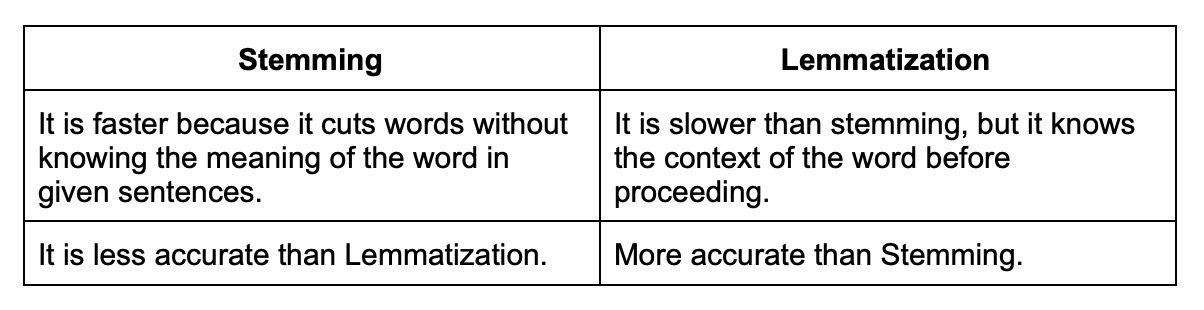#### 21.

What are the different types of text summarization?

There are two main types of text summarization:

Extraction-based: It does not take new phrases and words; instead, it uses the already existing phrases and words and presents only that. Extraction-based summarization ranks all the sentences according to the relevance and understanding of the text and presents you with the most important sentences.

Abstraction-based: It creates phrases and words, puts them together, and makes a meaningful word or sentence. Along with that, abstraction-based summarization adds the most important facts found in the text. It tries to find out the meaning of the whole text and presents the meaning to you.

#### 22.

What is the meaning of corpus in NLP?

Corpus in NLP refers to a large collection of texts. A corpus can be used for various tasks such as building dictionaries, developing statistical models, or simply for reading comprehension.

#### 23.

Explain binarizing of data.

Binarizing of data is the process of converting data features of any entity into vectors of binary numbers to make classifier algorithms more productive. The binarizing technique is used for the recognition of shapes, objects, and characters. Using this, it is easy to distinguish the object of interest from the background in which it is found.

#### 24.

What is perception and its types?

Perception is the process of interpreting sensory information, and there are three main types of perception: visual, auditory, and tactile.

Vision: It is used in the form of face recognition, medical imaging analysis, 3D scene modeling, video recognition, human pose tracking, and many more

Auditory: Machine Auditory has a wide range of applications, such as speech synthesis, voice recognition, and music recording. These solutions are integrated into voice assistants and smartphones.

Tactile: With this, machines are able to acquire intelligent reflexes and better interact with the environment.

#### 25.

Give some pros and cons of decision trees.

Decision trees have some advantages, such as being easy to understand and interpret, but they also have some disadvantages, such as being prone to overfitting.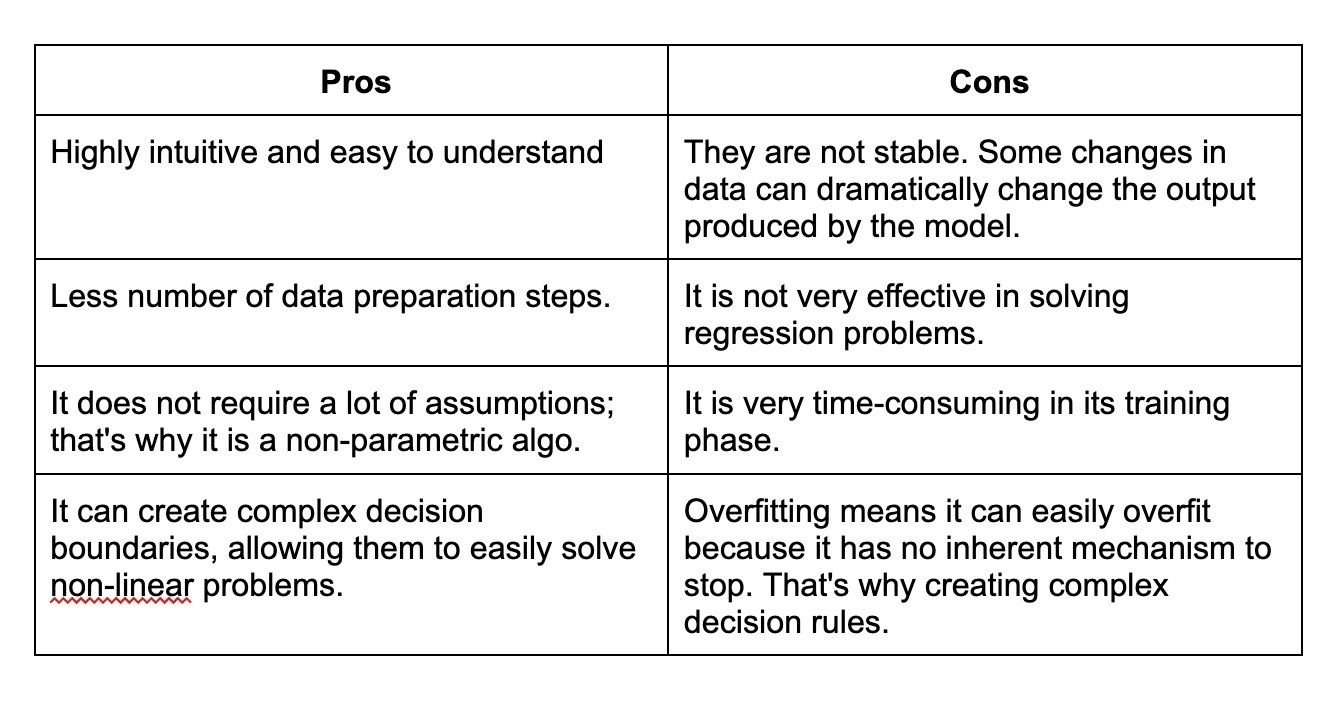#### 26.

Explain marginalization process.

The marginalization process is used to eliminate certain variables from a set of data, in order to make the data more manageable. In probability theory, marginalization involves integrating over a subset of variables in a joint distribution to obtain the distribution of the remaining variables. The process essentially involves "summing out" the variables that are not of interest, leaving only the variables that are desired.

#### 27.

What is the function of an artificial neural network?

An artificial neural network is a ML algorithm that is used to simulate the workings of the human brain. ANNs consist of interconnected nodes (also known as neurons) that process and transmit information in a way that mimics the behavior of biological neurons.

The primary function of an artificial neural network is to learn from input data, such as images, text, or numerical values, and then make predictions or classifications based on that data. ANNs can be used for a wide range of tasks, such as image recognition, natural language processing, and predictive analytics.

#### 28.

Explain cognitive computing and its types?

Cognitive computing is a subfield of AI that focuses on creating systems that can mimic human cognition and perform tasks that require human-like intelligence. The primary goal of cognitive computing is to enable computers to interact more naturally with humans, understand complex data, reason, learn from experience, and make decisions autonomously.

There is no strict categorization of cognitive computing types; however, the key capabilities and technologies associated with cognitive computing can be grouped as follows:

NLP: NLP techniques enable cognitive computing systems to understand, process, and generate human language in textual or spoken form.

Machine Learning: Machine learning is essential for cognitive computing, as it allows systems to learn from data, adapt, and improve their performance over time.

Computer Vision: Computer vision deals with the interpretation and understanding of visual information, such as images and videos. In cognitive computing, it is used to extract useful information from visual data, recognize objects, understand scenes, and analyze emotions or expressions.

#### 29.

Explain the function of deep learning frameworks.

Deep learning frameworks are software libraries and tools designed to simplify the development, training, and deployment of deep learning models. They provide a range of functionalities that support the implementation of complex neural networks and the execution of mathematical operations required for their training and inference processes. Some popular deep learning frameworks are TensorFlow, Keras, and PyTorch.

#### 30.

How are speech recognition and video recognition different?

Speech recognition and video recognition are two distinct areas within AI and involve processing and understanding different types of data. While they share some commonalities in terms of using machine learning and pattern recognition techniques, they differ in the data, algorithms, and objectives associated with each domain.

Speech Recognition focuses on the automatic conversion of spoken language into textual form. This process involves understanding and transcribing the spoken words, phrases, and sentences from an audio signal.

Video Recognition deals with the analysis and understanding of visual information in the form of videos. This process primarily involves extracting meaningful information from a series of image frames, such as detecting objects, recognizing actions, identifying scenes, and tracking moving objects.

#### 31.

What is the pooling layer on CNN?

A pooling layer is a type of layer used in a convolutional neural network (CNN). Pooling layers downsample the input feature maps by summary pooled areas. This reduces the dimensionality of the feature map and makes the CNN more robust to small changes in the input.

#### 32.

What is the purpose of Boltzmann machine?

Boltzmann machines are a type of energy-based model which learn a probability distribution by simulating a system of diverging and converging nodes. These nodes act like neurons in a neural network, and can be used to build deep learning models.

#### 33.

What do you mean by regular grammar?

Regular grammar is a type of grammar that specifies a set of rules for how strings can be formed from a given alphabet. These rules can be used to generate new strings or to check if a given string is valid.

#### 34.

How do you obtain data for NLP projects?

There are many ways to obtain data for NLP projects. Some common sources of data include texts, transcripts, social media posts, and reviews. You can also use web scraping and other methods to collect data from the internet.

#### 35.

Explain regular expression in layman’s terms.

Regular expressions are a type of syntax used to match patterns in strings. They can be used to find, replace, or extract text. In layman's terms, regular expressions are a way to describe patterns in data. They are commonly used in programming, text editing, and data processing tasks to manipulate and extract text in a more efficient and precise way.

#### 36.

How is NLTK different from spaCy?

Both NLTK and spaCy are popular NLP libraries in Python, but they have some key differences:

NLTK is a general-purpose NLP library that provides a wide range of tools and algorithms for basic NLP tasks such as tokenization, stemming, and part-of-speech tagging. NLTK also has tools for text classification, sentiment analysis, and machine translation. In contrast, spaCy focuses more on advanced NLP tasks such as named entity recognition, dependency parsing, and semantic similarity.

spaCy is generally considered to be faster and more efficient than NLTK due to its optimized Cython-based implementation. spaCy is designed to process large volumes of text quickly and efficiently, making it well-suited for production environments.

#### 37.

Name some best tools useful in NLP.

There are several powerful tools and libraries available for Natural Language Processing (NLP) tasks, which cater to various needs like text processing, tokenization, sentiment analysis, machine translation, among others. Some of the best NLP tools and libraries include:

NLTK: NLTK is a popular Python library for working with human language data. It provides easy-to-use interfaces to over 50 corpora and lexical resources, along with text processing libraries for classification, tokenization, stemming, tagging, parsing, and more.

spaCy: spaCy is a modern, high-performance, and industry-ready NLP library for Python. It offers state-of-the-art algorithms for fast and accurate text processing, and includes features like part-of-speech tagging, named entity recognition, dependency parsing, and word vectors.

Gensim: Gensim is a Python library designed for topic modeling and document similarity analysis. It specializes in unsupervised semantic modeling and is particularly useful for tasks like topic extraction, document comparison, and information retrieval.

OpenNLP: OpenNLP is an open-source Java-based NLP library that provides various components such as tokenizer, sentence segmenter, part-of-speech tagger, parser, and named entity recognizer. It is widely used for creating natural language processing applications.

#### 38.

Are chatbots derived from NLP?

Yes, chatbots are derived from NLP. NLP is used to process and understand human language so that chatbots can respond in a way that is natural for humans.

#### 39.

What is embedding and what are some techniques to accomplish embedding?

Embedding is a technique to represent data in a vector space so that similar data points are close together. Some techniques to accomplish embedding are word2vec and GloVe.

Word2vec: It is used to find similar words which have similar dimensions and, consequently, help bring context. It helps in establishing the association of a word with another similar meaning word through the created vectors.

GloVe: It is used for word representation. GloVe is developed for generating word embeddings by aggregating global word-word co-occurrence matrices from a corpus. The result shows the linear structure of the word in vector space.

### Tired of interviewing candidates to find the best developers?

Hire top vetted developers within 4 days.

### Intermediate Artificial Intelligence interview questions and answers

#### 1.

Why do we need activation functions in neural networks?

Activation functions play a vital role in neural networks, serving as a non-linear transformation applied to the output of a neuron or node. They determine the output of a neuron based on the weighted sum of its inputs, introducing non-linearity into the network. The inclusion of activation functions allows neural networks to model complex, non-linear relationships in the data.

#### 2.

Gradient descent is a popular optimization algorithm that is used to find the minimum of a function iteratively. It's widely used in machine learning and deep learning for training models by minimizing the error or loss function, which measures the difference between the predicted and actual values.

#### 3.

What is the purpose of data normalization?

Data normalization is a pre-processing technique used in machine learning and statistics to standardize and scale the features or variables in a dataset. The purpose of data normalization is to bring different features or variables to a common scale, which allows for more accurate comparisons and better performance of learning algorithms.

The main purposes of data normalization are:

Improving model performance: Some machine learning algorithms, like gradient-based optimization methods or distance-based classifiers, are sensitive to the feature scale.

Ensuring fair comparison: Normalization brings all features to a comparable range, mitigating the effect of different magnitudes or units of measurement, and ensuring that each feature contributes equally to the model's predictions.

Faster convergence: Gradient-based optimization algorithms can converge faster when data are normalized, as the search space becomes more uniformly scaled and the gradients have a more consistent magnitude.

Reducing numerical issues: Normalizing data can help prevent numerical issues like over- or underflow that may arise when dealing with very large or very small numbers during calculations.

#### 4.

Name some activation functions.

Some common activation functions include sigmoid, tanh, and ReLU.

Sigmoid: Maps the input to a value between 0 and 1, allowing for smooth gradient updates. However, it suffers from the vanishing gradient problem and is not zero-centered.

Tanh: Maps the input to a value between -1 and 1, providing a zero-centered output. Like the sigmoid function, it can also suffer from the vanishing gradient problem.

ReLU (Rectified Linear Unit): Outputs 0 for negative input values and retains the input for positive values. It helps alleviate the vanishing gradient problem and has faster computation time, but the output is not zero-centered and can suffer from the dying ReLU issue.

#### 5.

Briefly explain data augmentation.

Data augmentation is a technique used to increase the amount of data available for training a machine learning model. This is especially important for deep learning models, which require large amounts of data to train.

#### 6.

What is the Swish function?

The Swish function is an activation function. It is a smooth, non-linear, and differentiable function that has been shown to outperform some of the traditional activation functions, like ReLU, in certain deep learning tasks.

#### 7.

Explain forward propagation and backpropagation.

Forward propagation is the process of computing the output of a neural network given an input. Forward propagation involves passing an input through the network, layer by layer, until the output is produced. Each layer applies a transformation to the output of the previous layer using a set of weights and biases. The activation function is applied to the transformed output, producing the final output of the layer.

On the other hand, backpropagation is the process of computing the gradient of the loss function with respect to the weights of the network. It is used to update the weights and biases of the network during the training process. It involves calculating the gradient of the loss function with respect to each weight and bias in the network. The gradient is then used to update the weights and biases using an optimization algorithm such as gradient descent.

#### 8.

What is classification and its benefits?

Classification is a type of supervised learning task in machine learning and statistics, where the objective is to assign input data points to one of several predefined categories or labels. In a classification problem, the model is trained on a dataset with known labels and learns to predict the category to which a new, unseen data point belongs. Examples of classification tasks include spam email detection, image recognition, and medical diagnosis.

Some benefits of classification include:

Decision-making: Classification models can help organizations make informed decisions based on patterns and relationships found in the data.

Pattern recognition: Classification algorithms are capable of identifying and learning complex patterns in data, enabling them to predict the category of new inputs accurately.

Anomaly detection: Classification models can be used to detect unusual or anomalous data points that don't fit the learned patterns.

Personalization and recommendation: Classification models can be used to tailor content and recommendations to individual users, enhancing user experiences and increasing engagement.

#### 9.

What is a convolutional neural network?

Convolutional neural networks are a type of neural network that is well-suited for image classification tasks. In classification, the model learns to classify input data into one or more predefined classes or categories based on the features of the data. There are various benefits of classification, and it has numerous practical applications in different fields, such as:

Object Recognition: It is used in image and speech recognition to identify objects, faces, or voices.

Sentiment Analysis: It helps understand the polarity of textual data, which can be used to gauge customer feedback, opinions, and emotions.

Email Spam Filtering: It can be used to classify emails into a spam or non-spam categories to improve email communication.

#### 10.

Explain autoencoders and its types.

Autoencoders are a type of neural network that is used for dimensionality reduction. The different types of autoencoders include Denoising, Sparse, Undercomplete, etc.

Denoising Autoencoder: It is used to achieve good representation, meaning it can be obtained robustly from a corrupted input, which will be useful for recovering the corresponding clean input.

Sparse Autoencoder: This has a sparsity penalty, a value close to zero but not exactly zero. It is applied on the hidden layer in addition to the reconstruction error, which prevents overfitting.

Undercomplete Autoencoder: This does not need any regularization because they maximize the probability of data rather than copying the input to the output.

#### 11.

State fuzzy approximation theorem.

Fuzzy approximation theorem states that a function can be approximated as closely as desired using a combination of fuzzy sets. The theorem states that any continuous function can be represented as a weighted sum of linear functions, where the weights are fuzzy sets that capture the input variables' uncertainty.

#### 12.

What are the main components of LSTM?

LSTM stands for Long Short-Term Memory. It is a neural network architecture that is used for modeling time series data. LSTM has three main components:

The forget gate: This gate decides how much information from the previous state is to be retained in the current state.

The input gate: This gate decides how much new information from the current input is to be added to the current state.

The output gate: This gate decides what information from the current state is to be output.

#### 13.

Give some benefits of transfer learning.

Transfer learning is a machine learning technique where you use knowledge from one domain and apply it to another domain. This is usually done to accelerate the learning process or to improve performance.

There are several benefits of transfer learning:

Learn from smaller datasets: If you have a small dataset, you can use transfer learning to learn from a larger dataset in the same domain. This will help you to build better models.

Learn from different domains: You can use transfer learning to learn from different domains. For example, if you want to build a computer vision model, you can use knowledge from the medical domain.

Better performance: Transfer learning can help you to improve the performance of your models and apply it on other domains to build better models.

Pre-trained models: If you use a pre-trained model, you can save time and resources. This is because you don’t have to train the model from scratch.

Use of fine-tune models: You can fine-tune models using transfer learning. Also, you can adapt the model to your specific needs.

#### 14.

Explain the importance of cost/loss function.

The cost/loss function is an important part of machine learning that maps a set of input parameters to a real number that represents the cost or loss. The cost/loss function is used for optimization problems. The goal of optimization is to find the set of input parameters that minimize the cost/loss function.

#### 15.

Define the following terms - Epoch, Batch, and Iteration?

Epoch, batch, and iteration are all important terms in machine learning. Epoch refers to the number of times the training dataset is used to train the model; Batch refers to the number of training samples used in one iteration; Iteration is the number of times the training algorithm is run on the training dataset.

#### 16.

Explain dropouts.

Dropout is a method used to prevent the overfitting of a neural network. It refers to dropping out some neural network units. The process is similar to that of natural reproduction, where distinct genes combine to produce offspring while the other genes are dropped out instead of strengthening their co-adaptation.

#### 17.

As more layers are added and the distance from the final layer increases, backpropagation is not as helpful in sending information to the lower layers. As a result, the information is sent back, and the gradients start disappearing and becoming small in relation to network weights. These disappearing gradients are known as vanishing gradients.

#### 18.

Explain the function of batch Gradient Descent.

Batch gradient descent is an optimization algorithm that calculates the gradient of the cost function with respect to the weights of the model for each training batch. The weights are updated in the direction that decreases the cost function.

#### 19.

What is an Ensemble learning method?

Ensemble learning is a method of combining multiple models to improve predictive accuracy. These methods usually cost more to train but can provide better accuracy than a single model.

#### 20.

What are some drawbacks of machine learning?

One of the biggest drawbacks of Machine learning is that it can be biased if the data used to train the algorithm is not representative of the real world. For example, if an algorithm is trained using data that is mostly from one gender or one race, it may be biased against other genders or races.

Here are some other disadvantages of Machine Learning:

• Possibility of high Error
• Algorithm selection
• Data acquisition
• Time and space
• High production costs
• Lacking the skills to innovate

#### 21.

Explain Sentimental analysis in NLP?

Sentiment analysis is the process of analyzing text to determine the emotional tone of the text in NLP. This can be helpful in customer service to understand how customers are feeling, or in social media to understand the general public sentiment about a topic.

#### 22.

What is BFS and DFS algorithm?

Breadth-First Search (BFS) and Depth-First Search (DFS) are two algorithms used for graph traversal. BFS algorithm starts from the root node (or any other selected node) and visits all the nodes at the same level before moving to the next level.

On the other hand, DFS algorithm starts from the root node (or any other selected node) and explores as far as possible along each branch before backtracking.

#### 23.

Explain the difference between supervised and unsupervised learning.

Supervised learning involves training a model with labeled data, where both input features and output labels are provided. The model learns the relationship between inputs and outputs to make predictions for unseen data. Common supervised learning tasks include classification and regression.

Unsupervised learning, on the other hand, uses unlabeled data where only input features are provided. The model seeks to discover hidden structures or patterns in the data, such as clusters or data representations. Common unsupervised learning tasks include clustering, dimensionality reduction, and anomaly detection.

#### 24.

What is the text extraction process?

Text extraction is the process of extracting text from images or other sources. This can be done with OCR (optical character recognition) or by converting the text to a format that can be read by a text-to-speech system.

#### 25.

What are some disadvantages of linear models?

Here are some disadvantages of using linear models -

1. They can be biased if the data used to train the model is not representative of the real world.

2. Linear models can also be overfit if the data used to train the model is too small.

3. Linear models assume a linear relationship between the input features and the output variable, which may not hold in reality. This can lead to poor predictions and decreased model performance.

#### 26.

Mention methods for reducing dimensionality.

Artificial intelligence interview questions like this can be easy and difficult at the same time as you may know the answers but not on the tip of your tongue. Hence, a quick refresher can help a lot. Reducing dimensionality refers to the reduction of the number of random variables. This can be achieved by different techniques including principal component analysis, low variance filter, missing values ratio, high correlation filter, random forest, and others.

#### 27.

Explain cost function.

This is a popular AI interview question. A cost function is a scalar function that helps to identify how wrong an AI model is with regard to its ability to determine the relationship between X and Y. In other words, it tells us the neural network’s error factor.

The neural network works better when the cost function is lower. For instance, it takes the output predicted by the neural network and the actual output and then computes how incorrect the model was in its prediction.

So, the cost function will give a lower number if the predictions don’t differ too much from the actual values and vice-versa

#### 28.

Mention hyper-parameters of ANN.

The hyper-parameters of ANN are as follows:

Learning rate: It refers to the speed with which the network gets familiar with its parameters

Momentum: This parameter enables coming out of the local minima and smoothening jumps during gradient descent

The number of epochs: This parameter refers to the number of times the whole training dataset is fed to the network during training. One must increase the number of epochs until a decrease in validation accuracy is noticed, even if there is an increase in training accuracy, which is called overfitting.

Number of hidden layers: This parameter specifies the number of layers between the input and output layers.

Number of neurons in each hidden layer: This parameter specifies the number of neurons in each hidden layer.

Activation functions: Activation functions are responsible for determining a neuron's output based on the weighted sum of its inputs. Widely used activation functions include Sigmoid, ReLU, Tanh, and others.

#### 29.

Explain intermediate tensors. Do sessions have a lifetime?

Intermediate tensors are temporary data structures in a computational graph that store intermediate results when executing a series of operations in Artificial Intelligence, particularly in deep learning frameworks. These tensors represent the values produced during the forward pass of a neural network while processing input data before reaching the final output.

Yes, sessions have a lifetime, which starts when the session is created and ends when the session is closed or the script is terminated. In TensorFlow 1.x, sessions were used to execute and manage operations in a computational graph. A session allowed the allocation of memory for tensor values and held necessary resources to execute the operations. In TensorFlow 2.x, sessions and computational graphs have been replaced with a more dynamic and eager execution approach, allowing for simpler and more Pythonic code.

#### 30.

Explain Exploding variables.

Exploding variables are a phenomenon in which the magnitude of a variable grows rapidly over time, often leading to numerical instability and overflow errors. This can happen when a variable is repeatedly multiplied or divided by a value that is greater than 1 or less than -1. As a result, the variable's value grows exponentially or collapses to zero, causing computational problems.

#### 31.

Is it possible to build a deep learning model only using linear regression?

Linear regression is a basic tool in statistical learning, but it cannot be used to build a deep learning model. Deep learning models require non-linear functions to learn complex patterns in data.

#### 32.

What is the function of Hyperparameters ?

Hyperparameters are parameters that are not learned by the model. They are set by the user and used to control the model's behavior.

#### 33.

What is Artificial Super Intelligence (ASI)?

An Artificial Super Intelligence system is not one that has been achieved yet. Also known as Super AI, it is a hypothetical system that can surpass human intelligence and execute any task better than a human. The concept of ASI suggests that such an AI can exceed all human intelligence. It can even take complex decisions in harsh conditions and think just like a human would, or even better, develop emotional, sensible relationships.

#### 34.

What is overfitting, and how can it be prevented in an AI model?

Overfitting occurs when a model learns the training data too well, including capturing noise and random fluctuations. This often results in a model that performs poorly on unseen or validation data. Techniques to prevent overfitting include:

• Regularization (L1 or L2)
• Early stopping
• Cross-validation
• Using more training data
• Reducing model complexity

#### 35.

What is the role of pipeline for Information extraction (IE) in NLP?

Pipelines are used in information extraction to sequentially apply a series of processing steps to input data. This allows for efficient data processing and helps avoid errors.

#### 36.

What is the difference between full listing hypothesis and minimum redundancy hypothesis?

Full listing hypothesis states that all possible values of a variable should be listed in the data dictionary. Minimum redundancy hypothesis states that all values of a variable should be listed in the data dictionary, but that only the most important values should be listed multiple times.

### Tired of interviewing candidates to find the best developers?

Hire top vetted developers within 4 days.

#### 1.

Mention the steps of the gradient descent algorithm.

The gradient descent algorithm helps in optimization and in finding coefficients of parameters that help minimize the cost function. The steps that help achieve this are as follows:

Step 1: Give weights (x,y) random values and then compute the error, also called Sum of Squares Error (SSE).

Step 2: Compute the gradient or the change in SSE when you change the value of the weights (x,y) by a small amount. This step helps us identify the direction in which we must move x and y to minimize SSE.

Step 3: Adjust the weights with the gradients for achieving optimal values for the minimal SSE.

Step 4: Change the weights for predicting and calculating the new error. Step 5: Repeat steps 2 and 3 till the time making more adjustments stops producing significant error reduction.

These types of artificial intelligence interview questions help hiring managers properly guage a candidate’s expertise in this domain. Hence, you must thoroughly understand such questions and enlist all steps properly to move ahead.

#### 2.

Write a function to create one-hot encoding for categorical variables in a Pandas DataFrame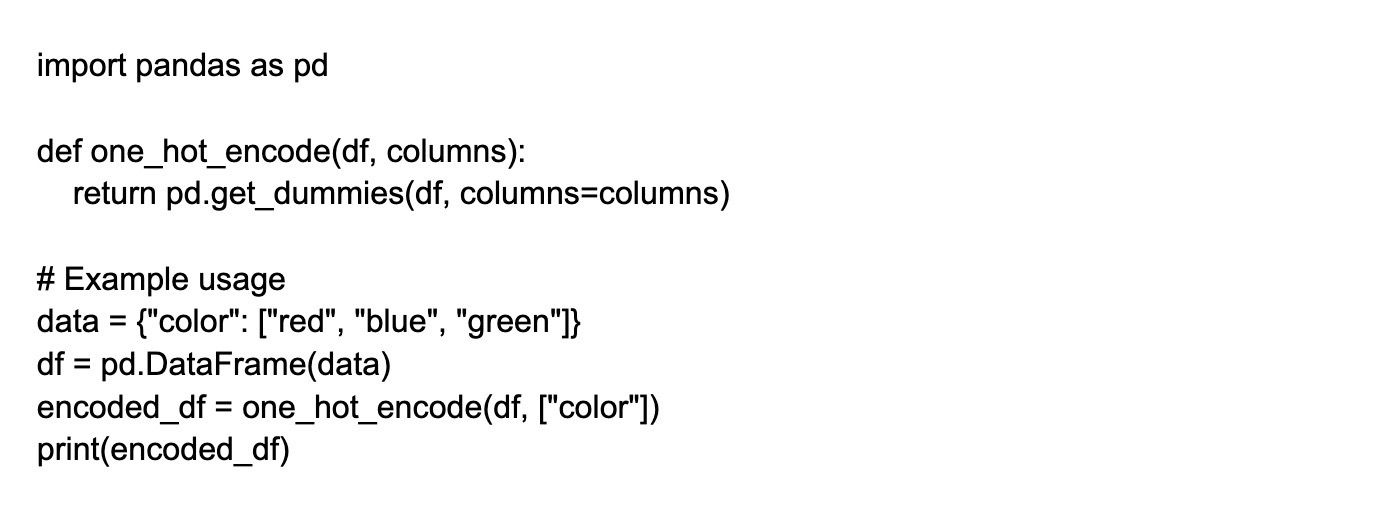#### 3.

Implement a function to calculate cosine similarity between two vectors.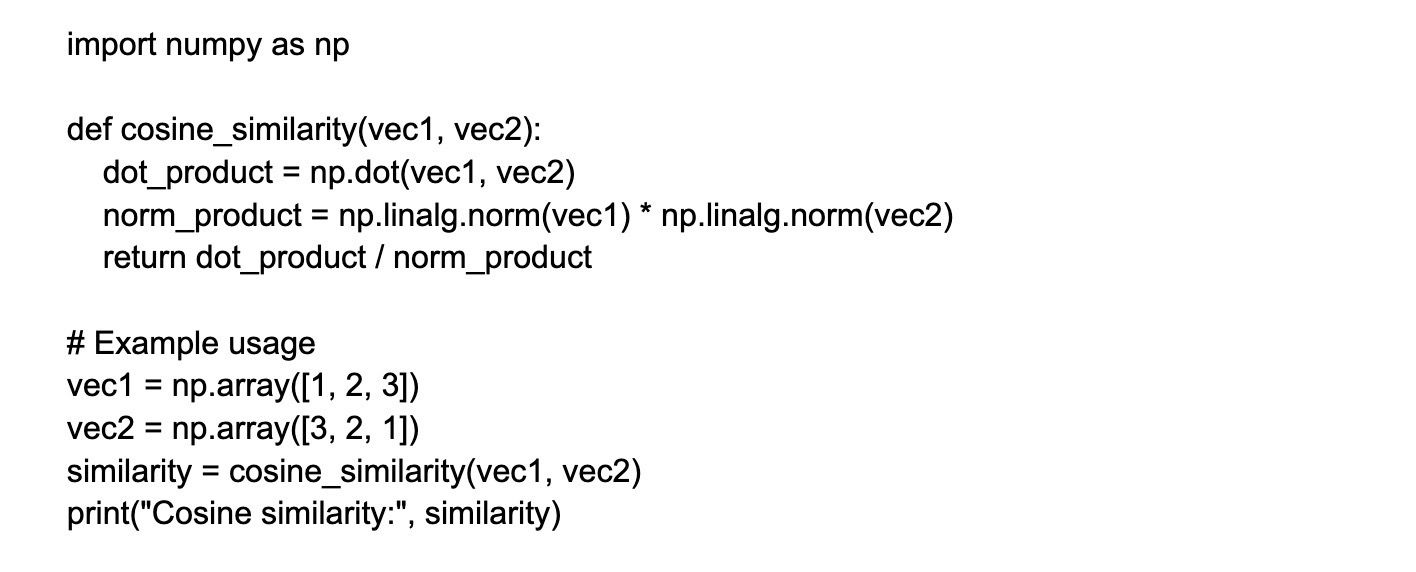#### 4.

How to handle an imbalance dataset?

There are a number of ways to handle an imbalanced dataset, such as using different algorithms, weighting the classes, or oversampling the minority class.

Algorithm selection: Some algorithms are better suited to handle imbalanced data than others. For example, decision trees and random forests tend to work well on imbalanced data, while algorithms like logistic regression or support vector machines may struggle.

Class weighting: By assigning higher weights to the minority class, you can make the algorithm give more importance to it during training. This can help prevent the algorithm from always predicting the majority class.

Oversampling: You can create synthetic samples of the minority class by randomly duplicating existing samples or generating new samples based on the existing ones. This can balance the class distribution and help the algorithm learn more about the minority class.

#### 5.

How do you solve the vanishing gradient problem in RNN?

The vanishing gradient problem is a difficulty encountered when training artificial neural networks using gradient-based learning methods. This problem is resolved by replacing the activation function of the network. You can use the Long Short-Term Memory (LSTM) network to solve the problem.

It has three gates called input, forgets, and output gates. Here forget gates constantly observe what information needs to be dropped going through the network. In this way, we have short and long-term memory. So, we can transfer the information through the network and retrieve it even at the last stage to identify the context of prediction.

#### 6.

Implement a function to normalize a given list of numerical values between 0 and 1.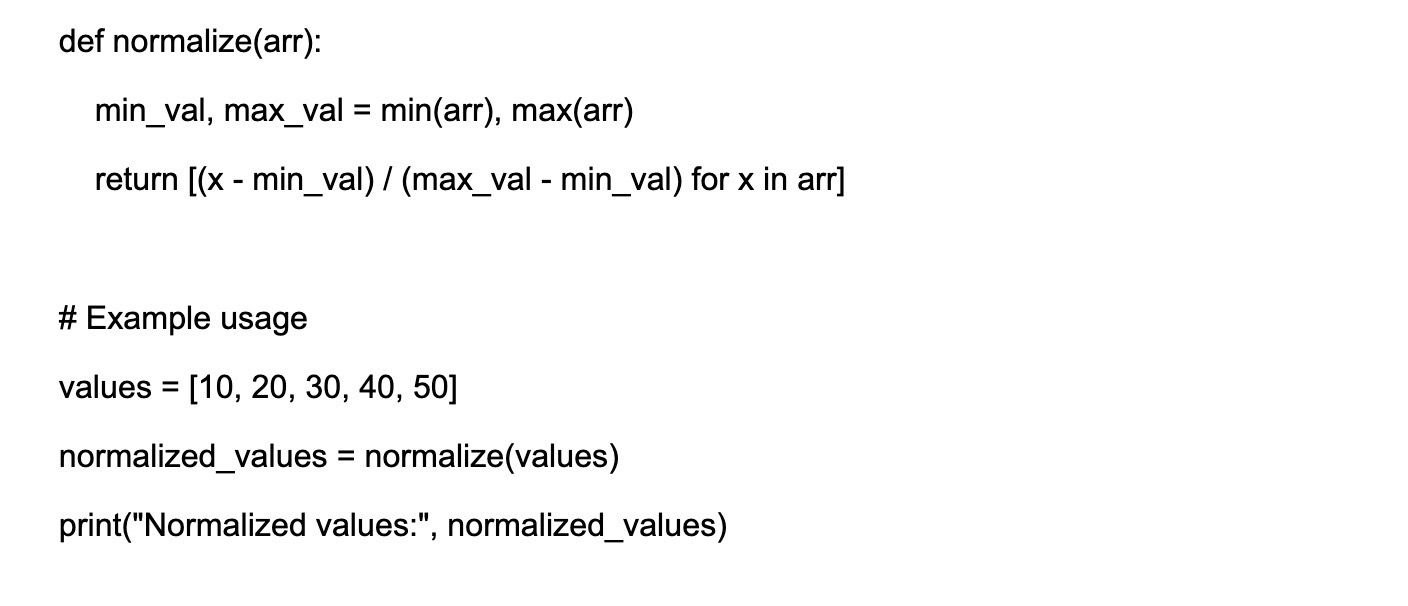#### 7.

Write a Python function to sort a list of numbers using the merge sort algorithm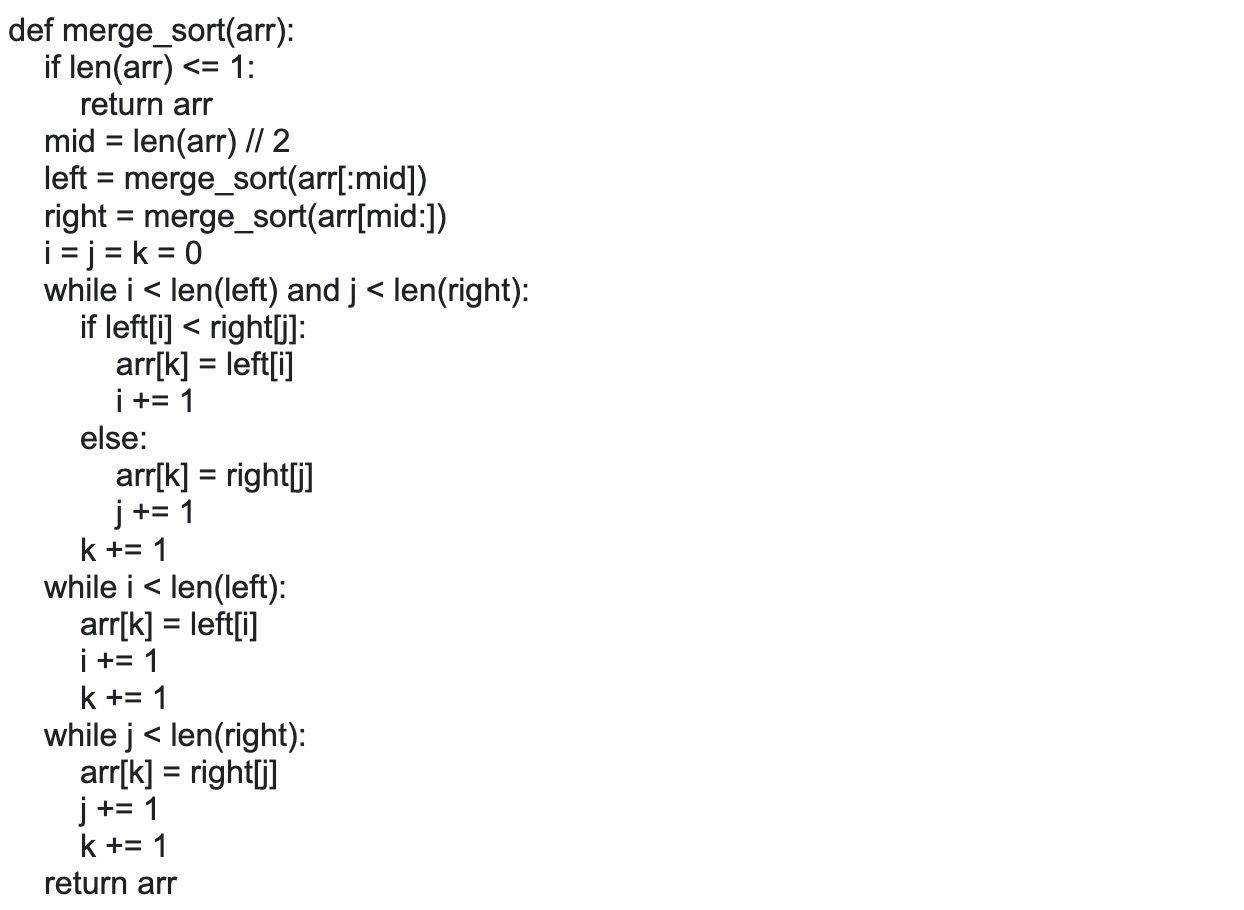#### 8.

Explain the purpose of Sigmoid and Softmax functions.

Sigmoid and softmax functions are used in classification problems. Sigmoid maps values to a range of 0-1, which is useful for binary classification problems. Softmax maps values to a range of 0-1 and also ensures that all values sum to 1, which is useful for multi-class classification problems.

#### 9.

Implement a Python function to calculate the sigmoid activation function value for any given input.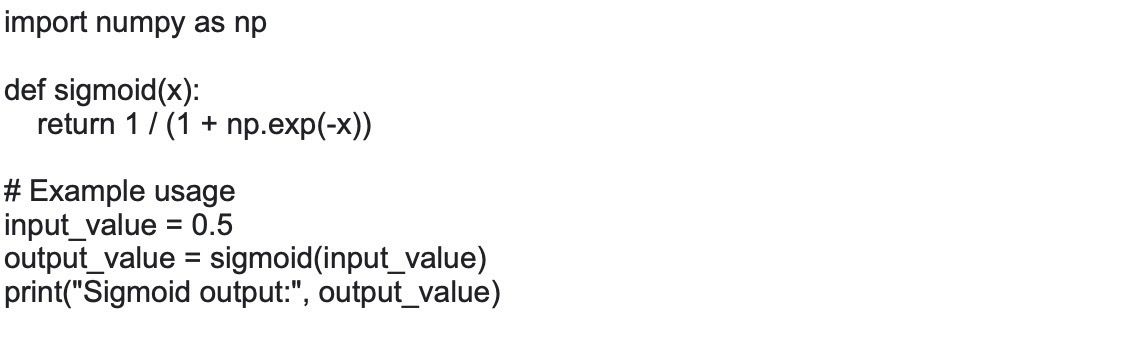#### 10.

Write a Python function to calculate R-squared (coefficient of determination) given true and predicted values.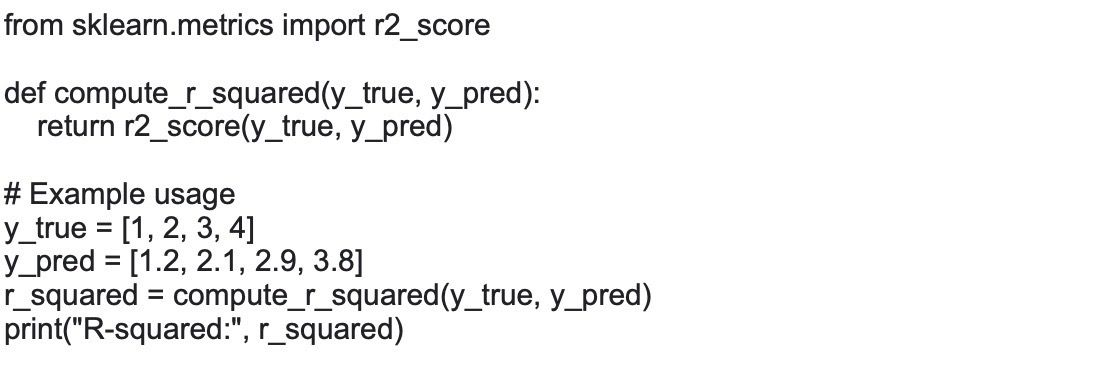#### 11.

Explain pragmatic analysis in NLP.

Pragmatic analysis is a process of analyzing text data in order to determine the speaker's intention. This is useful in many applications, such as customer service and market research. Here, the main focus is always on what was said to reconsider what is intentionally driving the various aspects of language that require real-world knowledge. It helps you to discover this intentional effect by applying a set of rules that characterize cooperative dialogues. Basically, it means abstracting the meaningful use of language in situations.

#### 12.

What is the difference between collaborative and content-based filtering?

Collaborative filtering is a method of making recommendations based on the likes and dislikes of a group of people, while Content-based filtering is a method of making recommendations based on the similarity of the content.

#### 13.

How is parsing achieved in NLP?

Parsing is the process of breaking down a string of text into smaller pieces, or tokens. This can be done using a regex, or a more sophisticated tool like a parser combinator. There are various techniques for parsing in NLP, including rule-based approaches, statistical approaches, and machine learning-based approaches. Some common parsing algorithms include the Earley parser, the CYK parser, and the chart parser. These algorithms use various methods such as probability models, tree-based representations, and context-free grammars to parse a text and identify its grammatical structure.

#### 14.

Implement a Python function to calculate the precision and recall of a binary classifier, given true positive, false positive, true negative, and false negative values.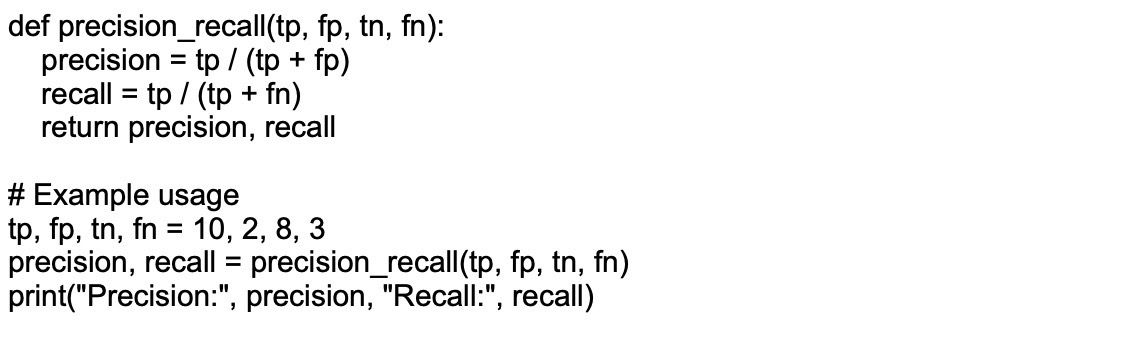#### 15.

What is Limited Memory? Explain with an example?

A human brain learns from its experiences or from the past experiences it has in its memory. Just like the human brain, Limited Memory Artificial Intelligence learns from past data already in the memory and makes decisions on their behalf. But this data is stored for some specific time, and they cannot add it to their information center. Self-Driving is one of the best technology examples of Limited Memory AI. Self Driving cars can store data during driving, like how many vehicles are moving around them, vehicle speed, and the traffic lights. From their experiences, they understand how to drive properly on the road in heavy and moderate traffic. Few companies are focused on these types of technologies.

#### 16.

Write a Python function to compute the Euclidean distance between two points.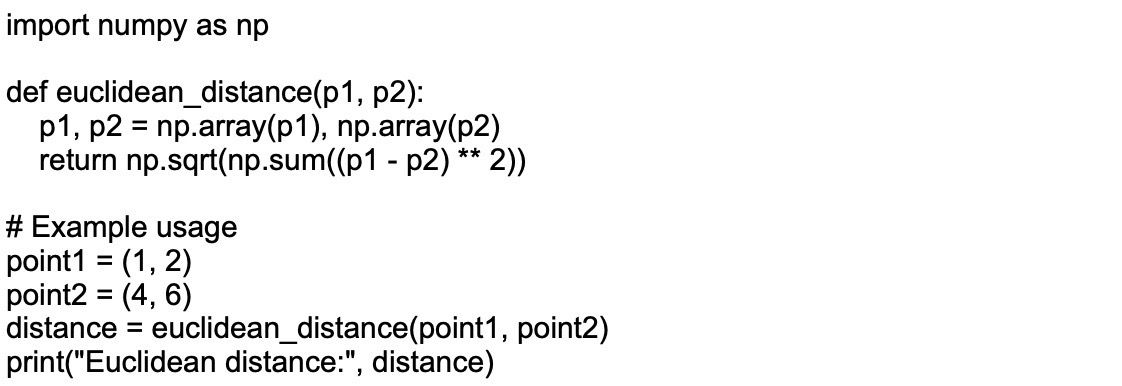#### 17.

Describe the differences between stochastic gradient descent (SGD) and mini-batch gradient descent.

Stochastic gradient descent (SGD) updates the model's weights using the gradient calculated from a single training example. It converges faster because of frequent weight updates; however, it can have a noisy convergence due to high variance in gradients.

Mini-batch gradient descent calculates the gradient using a small batch of training examples. It strikes a balance between the computational efficiency of batch gradient descent and the faster convergence of SGD. The noise in weight updates is reduced, leading to a more stable convergence.

#### 18.

Implement a function to calculate precision, recall, and F1-score given an input of actual and predicted labels.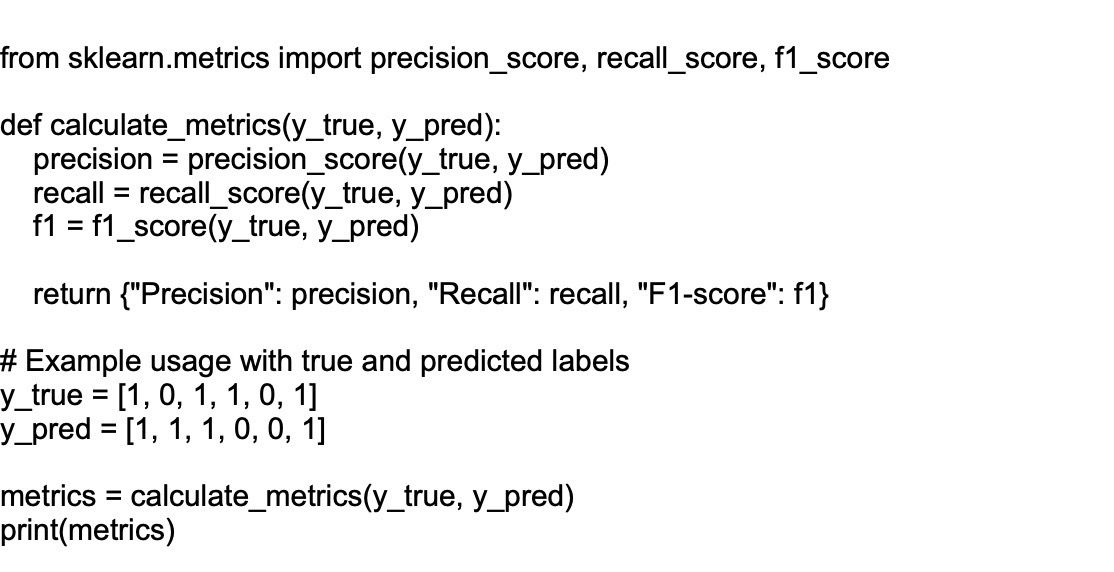#### 19.

How can you standardize data?

Data standardization is a technique that is mostly performed as a preprocessing step of developing ML models to formalize the range of features of an input data set.

Understanding data: You need to understand the distribution of your data to decide which standardization technique is appropriate. For example, if the data is normally distributed, you can use z-score normalization.

Choosing standardization technique: Standardization techniques such as z-score normalization, min-max scaling, and mean normalization can be used depending on the type of data.

Implementation: Standardizing data can be implemented using programming languages such as Python, and R, or tools such as Excel or a data automation platform.

Impact on model performance: Standardization can significantly impact the performance of machine learning models. Hence, it's important to standardize the data before feeding it into the model

#### 20.

How to implement Naive Bayes algo in Python ?

Here's a basic implementation of Naïve Bayes Classifier in Python using the scikit-learn library. This example demonstrates the process of loading a dataset, splitting it into training and testing sets, fitting the model, and calculating its accuracy.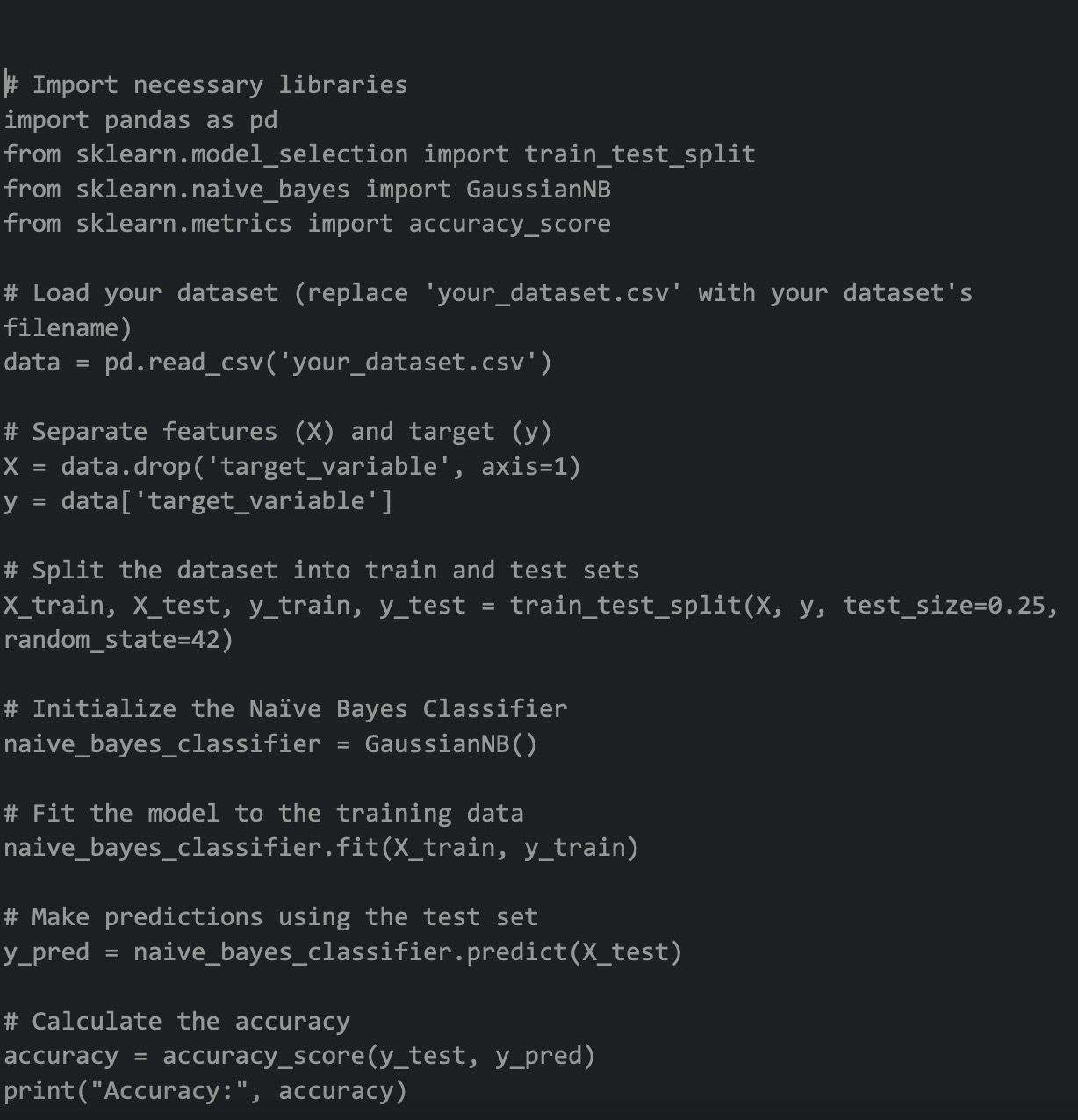#### 21.

Write a code to visualize data using Univariate plots.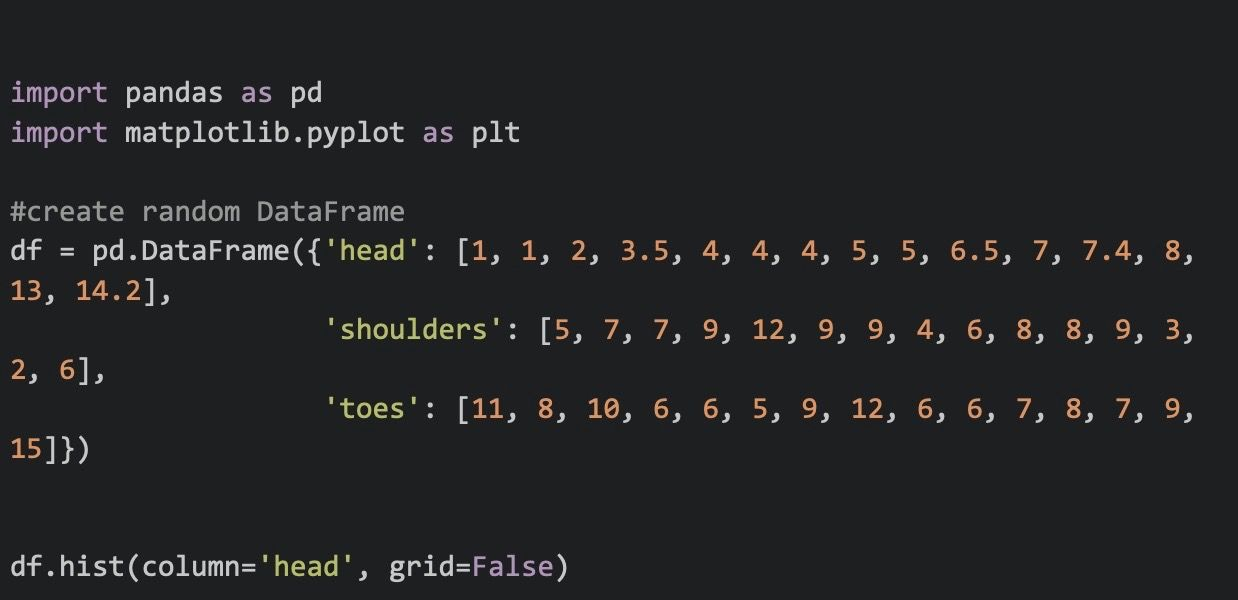#### 22.

How does information gain and entropy work in decision trees?

Entropy is unpredictability in the data; the more uncertainty, the higher the entropy will be. Entropy is used by information gain to make decisions. If the entropy is fewer, the information will be big.

Information gain is used in random forests and decision trees to decide the best split. Thus, the bigger the information gain, the better the split and the shorter the entropy. The entropy is used to calculate the information gain of a dataset before and after a split.

Entropy is the calculation of the probability of suspense in the data. The main purpose is to reduce entropy and increase information gain. The feature having the maximum information is considered essential by the algorithm and is used for training the model.

#### 23.

Write a code for random forest regression in Python.

Here's a basic implementation of the Random Forest Regressor in Python using the scikit-learn library. This example demonstrates the process of loading a dataset, splitting it into training and testing sets, fitting the model, and calculating the predictions.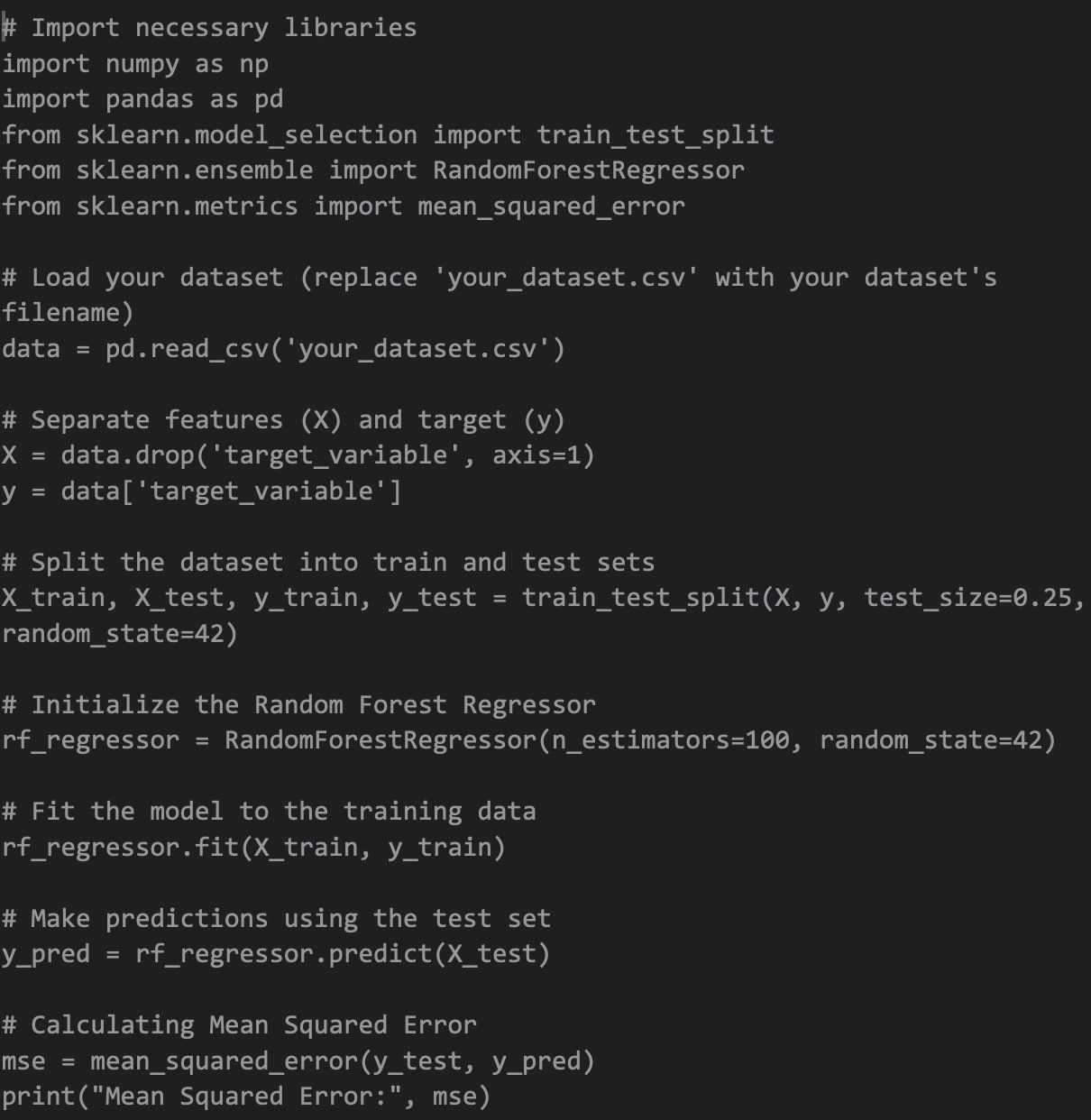#### 24.

Explain the use of kernel tricks?

Kernel tricks are a technique used in Artificial Intelligence, particularly in machine learning algorithms, to transform a non-linearly separable problem into a linearly separable one. They are commonly used in Support Vector Machines (SVMs) and other kernel-based algorithms for solving complex classification or regression tasks.

The main idea behind kernel tricks is to map the input data from a lower-dimensional space to a higher-dimensional space, in which the data points become linearly separable. This mapping is done using a mathematical function called the kernel function.

#### 25.

Write a code for K-nearest algorithm in Python.

Here's a basic implementation of the K-Nearest Neighbors (KNN) algorithm in Python using the scikit-learn library. This example demonstrates the process of loading a dataset, splitting it into training and testing sets, fitting the model, and calculating its accuracy.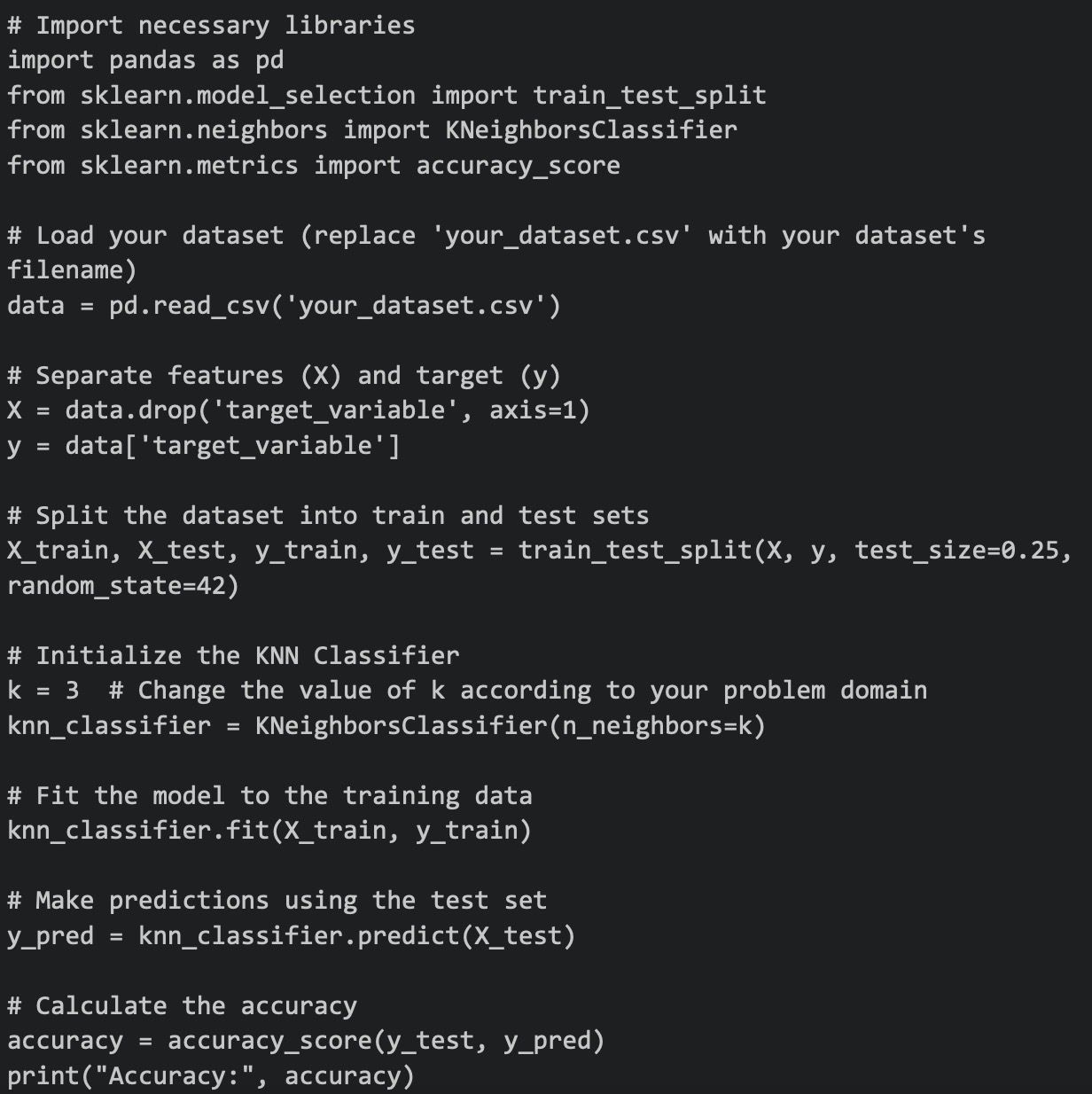#### 26.

How to calculate Gini coefficient?

The Gini coefficient formula is as follows:Here are a few steps using which you can calculate the Gini coefficient:

Organize the data into a table with the category head mentioned below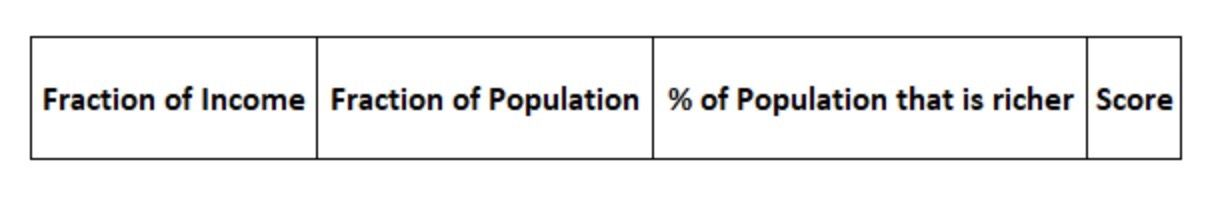All the rows must organize from the poorest to the richest. Fill the '% of Population that is richer' column by adding all terms in 'Fraction of Population' below that row. Calculate the Score for each of the rows. The formula for the Score is: Score = Fraction of Income * (Fraction of Population + 2 * % of Population that is richer). Next, add all the terms in the ‘Score’ column. Let us call it ‘Sum.’ Using the formula calculate the Gini coefficient: = 1 –Sum.

### Tired of interviewing candidates to find the best developers?

Hire top vetted developers within 4 days.

### Wrapping up

On this page, we have discussed the top 100 AI interview questions and answers that will be useful for both developers and recruiters in the hiring process. AI is a vast field and the questions are not limited to these; however, they are useful and cover the broad knowledge that an AI professional needs to be familiar with.

Moreover, if you’d like to skip this entire hiring process an onboard skilled AI developers or development teams, choose Turing and get it done seamlessly. Or, if you’re an AI developer looking for high-paying jobs with top US companies, click here to apply and get matched with elite US clients through Turing’s Talent Cloud.

###### Hire Silicon Valley-caliber Artificial Intelligence engineers at half the cost

Turing helps companies match with top-quality Artificial Intelligence engineers from across the world in a matter of days. Scale your engineering team with pre-vetted AI engineers at the push of a button.Hire from the top 1% developers worldwide

#### Hire remote developers

Tell us the skills you need and we'll find the best developer for you in days, not weeks.